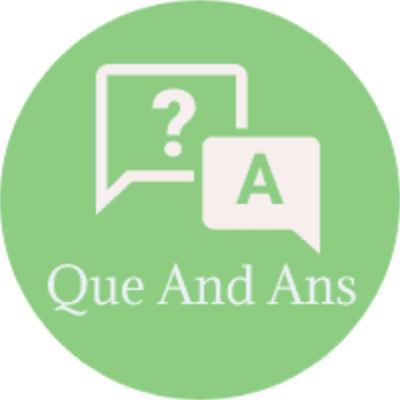Hello Friends Here in this section of Microprocessor Short Questions and Answers,We have listed out some of the important Short Questions with Answers which will help students to answer it correctly in their University Written Exam.

Lets have a look at below Lists of Short Descriptive type Questions that may be asked in this format in Written Exams.

1. How many memory locations can be addressed by a microprocessor with 14
The 8085 MPU with its 14-bit address is capable of addressing 214=16,384 (ie) 16K memory locations.

2. What is the function of the accumulator?
The accumulator is the register used to store the 8-bit data to perform the arithmetic and logical operations.

3. Define control bus?
This is single line that is generated by the MPU to provide timing of various operations.

4. What is a flag?
The data conditions, after arithmetic or logical operations, are indicated by setting or resetting the flip-flops called flags.

5. Why are the program counter and the stack pointer 16-bit registers?
Memory locations for the program counter and stack pointer have 16-dit address. So the PC and SP have 16-bit registers.

6. Define memory word?
The number of bits stored in a register is called a memory word.

7. Specify the number of registers and memory cells required in a 128 x 4
memory chip?
Number of registers=128
Memory cells required is 128 x 4=512

8. Explain the function of ALU and IO/M signals in the 8085 architecture?
The ALU signal goes high at the beginning of each machine cycle indicating the availability of the address on the address bus, and the signal is used to latch the low-order address bus. The IO/M signal is a status signal indicating whether the machine cycle is I/O or memory operation. The IO/M signal is combined with the RD and WR control signals to generate IOR, IOW, MEMW, MEMR.

9. If the 8085 adds 87H and 79H, specify the contents of the accumulator and the
status of the S, Z, and CY flag?
The sum of 87H and 79H=100H. Therefore, the accumulator will have 00H, and the flags will be S=0, Z=1, CY=1.

10. Write down the control and status signals?
Two control signals and three status signals
Control signals: RD and WR
Status signals: IO/M, S1, S2

11. Define machine cycle?
Machine cycle is defined, as the time required completing one operation of accessing memory, I/O, or acknowledging an external request.

12. Define T-state?
T-state is defined as one subdivision of the operation of performed in one clock period.

13. What is a transparent latch? Why is it necessary to latch with output devices
such as LED’s?
A transparent latch is a flip-flop; its output changes according to input when the clock signal is high, and it latches the input on the trailing edge of the clock. The latch is necessary for output devices to return the result; otherwise the result will disappear.

14. Define instruction cycle?
Instruction cycle is defined, as the time required completing the execution of the instruction.

15.What is an instruction set?
The entire group of instructions, determines what functions the microprocessor can perform is called instruction set.

16. Give the functional categories of 8085 micro instructions?
A Z AC P CY

• Data transfer operations
• Arithmetic operations
• Logical operations
• Branching operations
• Machine control operations

17. Define the types of branching operations?
Jump: to test the conditions Call, Return, And Restart: Change the sequence of the program

18. Define two-byte instruction with one example?
In a 2-byte instruction, the first byte specifies the Opcode; the second byte specifies the operand.
Example: Opcode operand MVI A, Data

19.What operation can be performed by using the instruction ADD A?
The instruction ADD a will add the content of the accumulator to itself; this is equivalent to multiplying by 2.

20. What is the machine control operations used in 8085 microprocessor?
HLT: Halt
NOP: No Operation

You may be interested in:
Microprocessor MCQs
Microprocessor 8086 MCQs
Microprocessor Online Tests
Microprocessor Tutorials